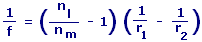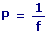Lensmaker Equation Calculator Enter value and click on calculate. Result will be displayed.f = Focal Point nl = Refractive Index of Lens Material nm = Refractive Index of Ambient Medium r1 = Radius of Curvature of the First Surface r2 = Radius of Curvature of the Second Surface P = Lens Power
 Enter your values: Radius of Curvature of the First Surface: Cm Radius of Curvature of the Second Surface: Cm Refractive Index of Lens Material: m Refractive Index of Ambient Medium: m Result: Focal Point: Cm Lens Power: Diopters

 Web www.calculatoredge.com
 Lensmaker Equation is used to determine whether a lens will behave as a converging or diverging lens based on the curvature of its faces and the relative indices of the lens material and the surrounding medium. This equation is used for determining the focal length of a thin lens (thickness = 0) with radii of curvature r1 and r2.

The focal length of a lens in air can be calculated from the lensmaker's equation. For a thin lens, the power is approximately the sum of the surface powers.

There are basically four factors that determine the focal length of a lens: the index of refraction of the lens, the index of refraction of the medium which forms the environment of the lens, the radius of the front surface of the lens, and the radius of the back surface of the lens. The equation that relates these quantities to the focal length of the lens is called the lensmaker's equation, since it gives the focal length in terms of quantities involved in the manufacture of the lens.

Lensmaker Equation is used to make Lenses.LINKSDISCLAIMERCONTACT US